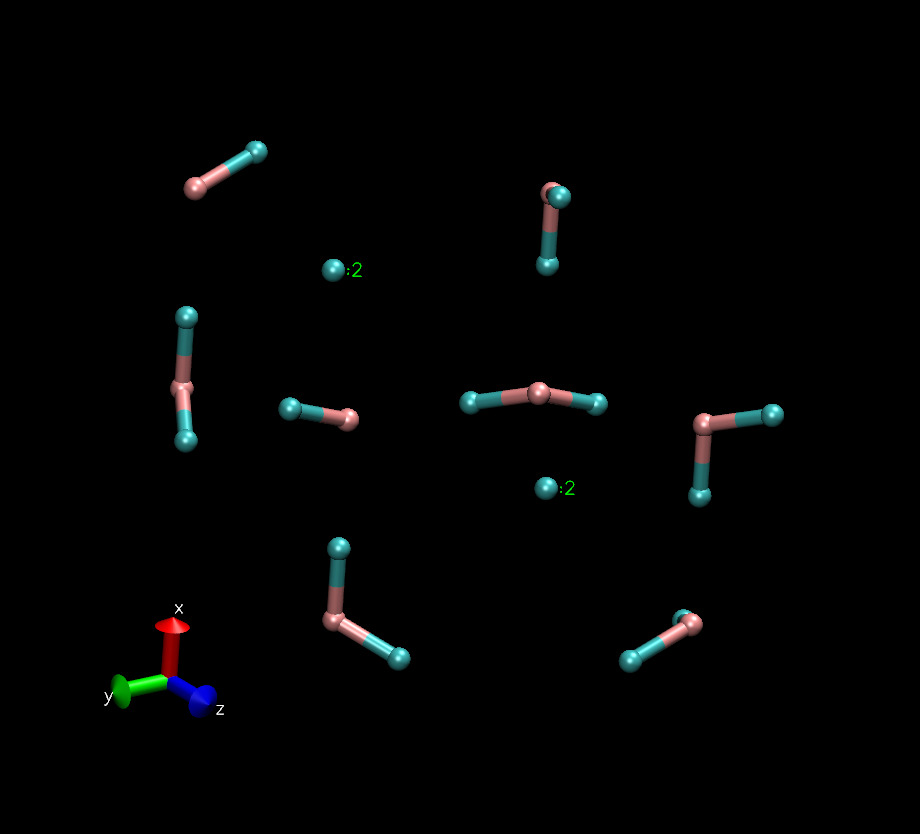# Problem with pair style interacting with neighbor list

I apologize in advance for the vagueness of this query as I really have very little idea what is happening. I am trying to error check my code against an ice crystal for which I know the correct gradients/energy etc. (Attached) For at least two of the 24 atoms the neighbor list appears to be incorrect. I suspect it is actually something in the way I am querying the neighbor list but really have no idea how, as every attempt was made to copy exactly other pair styles. While the problem occurs twice it is most noticeable with the last atom. When I compute the r distances of this atom to the rest in its neighbor I get the following list:

r = 8.405522

r = 5.660089

r = 5.568298

r = 6.483076

r = 5.120685

r = 8.242079

r = 8.753554

r = 8.404739

r = 9.464110

r = 4.829808

r = 4.662876

r = 4.010953

r = 8.245411

r = 7.475285

r = 6.222468

r = 5.602602

r = 4.763255

r = 4.423263

r = 4.205935

r = 3.664023

r = 3.874214

Conspicuously absent are the two atoms of primary interest one at 0.97Å and the other at either 1.59Å/1.75Å.

Any ideas would be appreciated.
thanks
matthew wander

pair_vmm.cpp (61.2 KB)

pair_vmm.h (5.05 KB)

waterbox_small_vmm.inp (681 Bytes)

waterbox_small_vmm.data (2.6 KB)

oh.vmm (3.34 KB)

waterbox_small.gjf (1.58 KB)

I apologize in advance for the vagueness of this query as I really have very
little idea what is happening. I am trying to error check my code against an
ice crystal for which I know the correct gradients/energy etc. (Attached)
For at least two of the 24 atoms the neighbor list appears to be incorrect.
I suspect it is actually something in the way I am querying the neighbor
list but really have no idea how, as every attempt was made to copy exactly
other pair styles. While the problem occurs twice it is most noticeable with
the last atom. When I compute the r distances of this atom to the rest in
its neighbor I get the following list:

r = 8.405522

r = 5.660089

r = 5.568298

r = 6.483076

r = 5.120685

r = 8.242079

r = 8.753554

r = 8.404739

r = 9.464110

r = 4.829808

r = 4.662876

r = 4.010953

r = 8.245411

r = 7.475285

r = 6.222468

r = 5.602602

r = 4.763255

r = 4.423263

r = 4.205935

r = 3.664023

r = 3.874214

Conspicuously absent are the two atoms of primary interest one at 0.97Å and
the other at either 1.59Å/1.75Å.

when i visualize your data file, there are two atoms with no close neighbors.
so it looks as if the neighbor lists are correct.

axel.